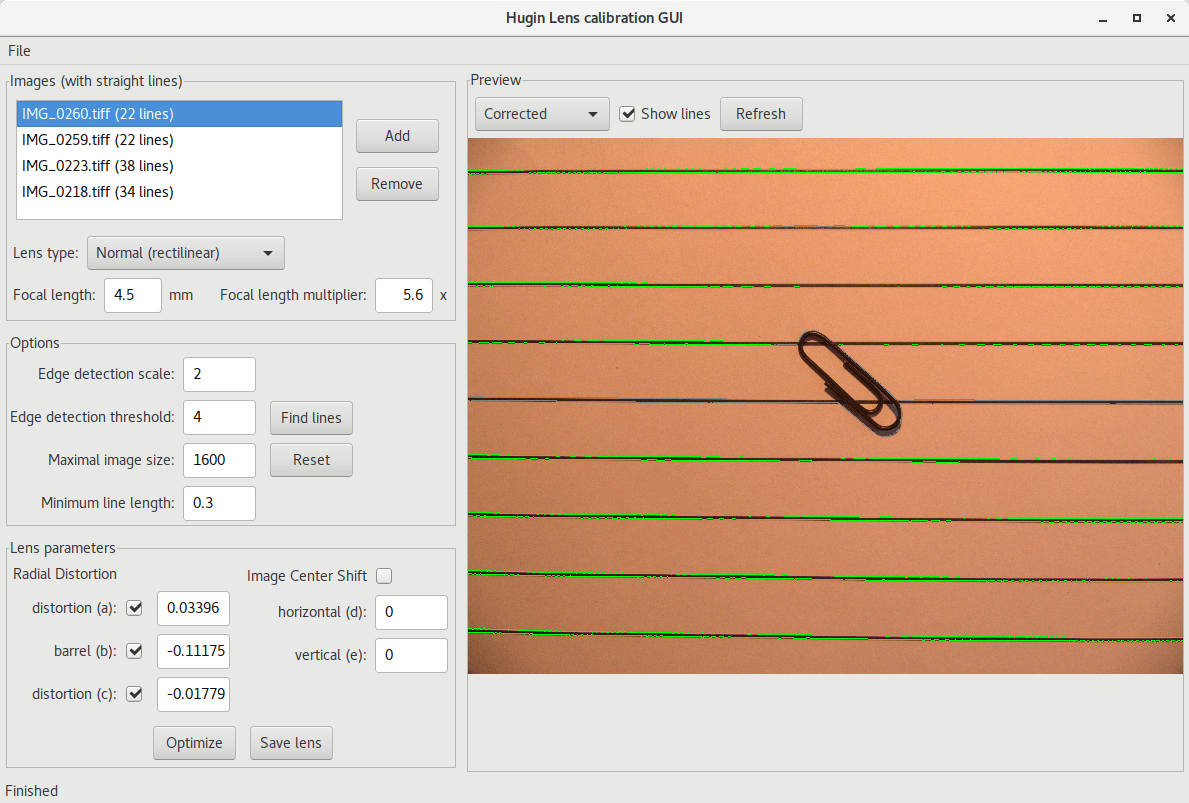# Lens calibration workflow for Canon PowerShot SX710HS

CHDK adds a RAW shooting option to many point and shoot cameras, including Canon PowerShot SX710HS. As the camera does not apply lens correction automatically for RAWs, one has to fix lens distortion when developing the RAWs. Lensfun is the go-to place for FOSS lens correction, however it did not have lens correction data for the SX710HS. So I’ve decided to calibrate the lens myself.

The Lensfun tutorial for lens calibration is quite cumbersome. It directs you go out and shoot some buildings with straight lines, and then manually track those lines in Hugin and extract the distortion parameters. Well, after searching a bit, I came up with what I think is a better workflow.

I’ve started by creating in Inkscape (using the Cartesian grid tool) and printing a page with regularly spaced horizontal lines. I’ve printed it on an A3 paper, but in hindsight A4 would have worked just the same and is more available. I’ve placed the paper on the floor, added some simple lightning and and placed a paperclip in the middle just to make focusing easier.

Next I’ve took several shoots at each focal length, having the printed lines parallel to the long side of the image. To make sure I’m covering the entire zoom range consistently, I’ve used my `set_zoom` script. I’ve taken calibration shoots in 0 (minimal focal lenght), 5, 10, 20, … ,100, 110 and 111 (maximal focal length) zoom steps. After taking all the shots, I’ve copied the DNGs (CHDK saved RAWs as DNGs) from the camera, and used `exiftool` to sort them into directories by focal length:

``\$ exiftool '-directory<\${FocalLength;}' *.DNG``

As we will use Hugin, for the calibration and Hugin does not support DNGs, we have to convert them to TIFFs.

``find -name "*.DNG" | xargs --verbose -P6 -I{} dcraw -t 0 -T {}``

The `-t 0` is used to remove the orientation info from the TIFFs. As I shoot the pictures with camera pointing straight down, that info is many times incorrect and messes up the calibration in Hugin.

Load each set of TIFFs of a given focal length into Hugin Lens Calibration GUI. Make sure the focal length and focal length multiplier are correct (for some reason Hugin was off by 0.01-0.02 sometimes), and use the “Find lines” button to automatically detect straight lines. Next optimize the radial distortion parameters. Those `a`, `b` and `c` parameters are the numbers Lensfun needs.Hugin Lens Calibration GUI, after line detection and optimization.

The next step is to create a Lensfun profile for the camera. The basic blocks are the `<camera>` tag and the `<lens>` tag. Both are mandatory, even thought the camera has a fixed lens. For each focal length we calibrated the lens for, we add a `<distortion>` tag, modifiying the `focal` and the `a`, `b ` and `c` parameters according to the values from the calibration GUI.

``````<lensdatabase version="1">
<camera>
<maker>Canon</maker>
<model>Canon PowerShot SX710 HS</model>
<model lang="en">PowerShot SX710 HS</model>
<mount>canonSX710HS</mount>
<cropfactor>5.6</cropfactor>
</camera>
<lens>
<maker>Canon</maker>
<model>Canon PowerShot SX710 HS & compatibles, with CHDK's DNG</model>
<model lang="en">fixed lens, with CHDK's DNG</model>
<model lang="de">festes Objektiv, mit CHDK-DNG</model>
<mount>canonSX710HS</mount>
<cropfactor>5.6</cropfactor>
<aspect-ratio>4:3</aspect-ratio>
<calibration>
<distortion model="ptlens" focal="4.5" a="0.03396" b="-0.11175" c="-0.01779" />
<distortion model="ptlens" focal="5.5" a="0.03289" b="-0.11253" c="0.02444" />
<distortion model="ptlens" focal="6.6" a="0.02218" b="-0.08388" c="0.03521" />
<distortion model="ptlens" focal="9.5" a="0.01211" b="-0.04143" c="0.02055" />
<distortion model="ptlens" focal="13.5" a="-0.0022" b="0.00264" c="-0.00558" />
<distortion model="ptlens" focal="18.2" a="-0.00212" b="0.00441" c="-0.00378" />
<distortion model="ptlens" focal="23.4" a="0.00328" b="-0.01242" c="0.01298" />
<distortion model="ptlens" focal="29.1" a="-0.00036" b="-0.00082" c="0.00209" />
<distortion model="ptlens" focal="36.2" a="0.00129" b="-0.00626" c="0.00705" />
<distortion model="ptlens" focal="46.3" a="0.00715" b="-0.02588" c="0.0244" />
<distortion model="ptlens" focal="46.3" a="0.00715" b="-0.02588" c="0.0244" />
<distortion model="ptlens" focal="62.9" a="0.00067" b="-0.00852" c="0.01085" />
<distortion model="ptlens" focal="92.0" a="-0.00306" b="0.00016" c="-0.00374" />
<distortion model="ptlens" focal="128.7" a="-0.0037" b="0.00365" c="-0.00582" />
<distortion model="ptlens" focal="135.0" a="0.00333" b="-0.02616" c="0.03388" />
</calibration>
</lens>
</lensdatabase>``````

The lens’ model name follows the Lensfun convention for CHDK. However, it makes auto-detection of the lens to fail in many application such as Darktable. You can workaround it by copying exactly the model name from the `<camera>` tag. The xml file itself should go in `~/.local/share/lensfun`.

The two pictures in the beginning of the post show some examples of uncorrected and corrected photos using the newly acquired calibration data.

This site uses Akismet to reduce spam. Learn how your comment data is processed.